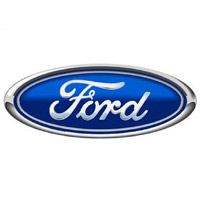“现代嘉年华”北京站启幕 惊喜不断

• 领界EV预售价18.28万元起 即将上市
• 骏铃轻量化车型上市
• 福特领界 1月份上市
• 捷途X90 1月13日上市
• 新奥迪A6L 1月15日上市
• 上汽大通G50 更多 >> 2月28日上市
• 新闻中心

设计成就梦想 与东风标致4008共赏巴黎时装周

新闻2019-10-21超过12878次关注

9月23日，引领全球设计风潮的2020春夏巴黎时装周大幕初启，此次时装周上，东风标致4008与搜狐时尚共同携手，直击巴黎时装周全球时尚顶级秀场，共同展示风领全球的时...

安全随时守护，2019款小蚂蚁伴你安心出行

新闻2019-10-21超过8923次关注

中国汽车行业经历数十年的高速发展，消费者对于汽车的需求也从“能开”升级到“好开”再升级到“好看又好开”，这也就是为什么2019款小蚂蚁一经上市就引发“城市潮...

东风标致全新一代2008申报图曝光

新闻2019-10-21超过11632次关注

中国江西网/江西头条新闻客户端讯近日，国家工信部官方网站公布了东风标致全新一代2008车型图片。这款标致为全球市场打造的全新SUV将于2019广州国际车展首次亮相。...

江淮康铃全面启动

新闻2019-10-21超过12336次关注

双十一——全球商业的狂欢日。随着网购狂欢节“双十一”的临近,国内各大快递企业已提前开始部署和优化车队运力。为保证这场狂欢盛宴的的极致体验,江淮康铃作为“四...

秦岚驾驭东风标致4008 邂逅巴黎白月光

新闻2019-10-21超过13209次关注

这是巴黎最美的季节，美得让人嫉妒、让人欲罢不能。塞纳河在醉人的秋色中从容淌过，左岸潮流时尚的繁华与右岸瑰丽多彩的风景，就像吵闹不休却又不离不弃的恋人，随...

哈弗2019年累计销量再度夺魁

新闻2019-10-21超过13427次关注

近日,“人气天王”周杰伦再现“神车技”登顶微博热搜,而在车市,同样有着一个“天王级”品牌以近50%的环比增长率,占据市场新高度,TA就是哈弗SUV。在刚刚公布的9月销...

热车排行

按类型 按车价
• 全部
• 微型
• 小型
• 紧凑型
• 中型
• 中大型
• 豪华型
• SUV
• MPV
•
• 郑州车市行情
郑州车商推荐东风悦达起亚河南普泽6S旗舰店

0371-86521188河南宇之林汽车销售服务有限公司

0371-55634444郑州市金裕通汽车销售服务有限公司

0371—63832666河南郑东天道长安福特4S旗舰店

0371-85333002郑州郑德宝汽车销售服务有限公司

4000663368-6268
我要维权
购车、修车遇到问题？专业律师在线，免费咨询维权！
﻿
• 快速找车
• 选择品牌
• 选择品牌
• A  奥迪
• A  阿斯顿·马丁
• A  阿尔法·罗密欧
• B  宝沃
• B  布加迪
• B  巴博斯
• B  保时捷
• B  宾利
• B  奔驰
• B  宝马
• B  本田
• B  别克
• B  标致
• B  比亚迪
• B  宝骏
• B  北汽制造
• B  北汽新能源
• B  北汽幻速
• B  北汽威旺
• B  北京汽车
• B  奔腾
• B  北汽绅宝
• C  长安
• C  长安商用
• C  长城
• C  昌河
• D  大众
• D  道奇
• D  DS
• D  东南
• D  东风风神
• D  东风风行
• D  东风小康
• D  东风风度
• D  东风
• F  福特
• F  丰田
• F  菲亚特
• F  法拉利
• F  福田
• F  福迪
• F  福汽启腾
• G  观致
• G  广汽传祺
• G  广汽吉奥
• G  GMC
• H  红旗
• H  汉腾汽车
• H  哈弗
• H  哈飞
• H  海格
• H  海马
• H  华颂
• H  黄海
• H  华泰
• H  恒天
• J  吉利汽车
• J  捷豹
• J  Jeep
• J  江淮
• J  江铃
• J  金杯
• J  九龙
• J  金旅
• K  凯翼
• K  凯迪拉克
• K  克莱斯勒
• K  科尼塞克
• K  卡威
• K  开瑞
• L  路虎
• L  林肯
• L  劳斯莱斯
• L  兰博基尼
• L  雷克萨斯
• L  铃木
• L  雷诺
• L  理念
• L  力帆
• L  莲花汽车
• L  猎豹
• L  路特斯
• L  陆风
• M  马自达
• M  MG
• M  MINI
• M  玛莎拉蒂
• M  摩根
• M  迈凯轮
• N  纳智捷
• O  欧宝
• O  讴歌
• O  欧朗
• Q  奇瑞
• Q  起亚
• Q  启辰
• R  日产
• R  荣威
• R  瑞麒
• S  三菱
• S  斯威汽车
• S  萨博
• S  smart
• S  斯柯达
• S  斯巴鲁
• S  思铭
• S  双龙
• S  上汽大通
• S  双环
• T  特斯拉
• T  腾势
• W  沃尔沃
• W  五菱汽车
• W  五十铃
• W  威兹曼
• W  威麟
• X  现代
• X  雪佛兰
• X  雪铁龙
• X  西雅特
• Y  一汽
• Y  英菲尼迪
• Y  英致
• Y  依维柯
• Y  野马汽车
• Y  永源
• Z  众泰
• Z  中华
• Z  中兴
• Z  知豆
• 选择车系
• 选择车系
• 车型对比
• 选择品牌
• 选择品牌
• A  奥迪
• A  阿斯顿·马丁
• A  阿尔法·罗密欧
• B  宝沃
• B  布加迪
• B  巴博斯
• B  保时捷
• B  宾利
• B  奔驰
• B  宝马
• B  本田
• B  别克
• B  标致
• B  比亚迪
• B  宝骏
• B  北汽制造
• B  北汽新能源
• B  北汽幻速
• B  北汽威旺
• B  北京汽车
• B  奔腾
• B  北汽绅宝
• C  长安
• C  长安商用
• C  长城
• C  昌河
• D  大众
• D  道奇
• D  DS
• D  东南
• D  东风风神
• D  东风风行
• D  东风小康
• D  东风风度
• D  东风
• F  福特
• F  丰田
• F  菲亚特
• F  法拉利
• F  福田
• F  福迪
• F  福汽启腾
• G  观致
• G  广汽传祺
• G  广汽吉奥
• G  GMC
• H  红旗
• H  汉腾汽车
• H  哈弗
• H  哈飞
• H  海格
• H  海马
• H  华颂
• H  黄海
• H  华泰
• H  恒天
• J  吉利汽车
• J  捷豹
• J  Jeep
• J  江淮
• J  江铃
• J  金杯
• J  九龙
• J  金旅
• K  凯翼
• K  凯迪拉克
• K  克莱斯勒
• K  科尼塞克
• K  卡威
• K  开瑞
• L  路虎
• L  林肯
• L  劳斯莱斯
• L  兰博基尼
• L  雷克萨斯
• L  铃木
• L  雷诺
• L  理念
• L  力帆
• L  莲花汽车
• L  猎豹
• L  路特斯
• L  陆风
• M  马自达
• M  MG
• M  MINI
• M  玛莎拉蒂
• M  摩根
• M  迈凯轮
• N  纳智捷
• O  欧宝
• O  讴歌
• O  欧朗
• Q  奇瑞
• Q  起亚
• Q  启辰
• R  日产
• R  荣威
• R  瑞麒
• S  三菱
• S  斯威汽车
• S  萨博
• S  smart
• S  斯柯达
• S  斯巴鲁
• S  思铭
• S  双龙
• S  上汽大通
• S  双环
• T  特斯拉
• T  腾势
• W  沃尔沃
• W  五菱汽车
• W  五十铃
• W  威兹曼
• W  威麟
• X  现代
• X  雪佛兰
• X  雪铁龙
• X  西雅特
• Y  一汽
• Y  英菲尼迪
• Y  英致
• Y  依维柯
• Y  野马汽车
• Y  永源
• Z  众泰
• Z  中华
• Z  中兴
• Z  知豆
• 选择车系
• 选择车系
• 选择车型
• 选择车型
• 意见反馈